### 2.2.3 Lifetime and Diffusion LengthSo far we looked at (perfect) semiconductors in perfect equilibrium. The density of holes and electrons was given by the type of the semiconductor (as signified by the band gap), the doping, and the temperature.The only other property of interest (introduced in passing) was the carrier mobility – a quantity that is determined in a comparatively complex way by properties of the semiconducting materials in question.The carrier density so far was constant and did not change in time – we perceived the system statically.

Concept of Life TimeIn reality, however, we have a dynamic equilibrium : Electron–hole pairs are generated all the time and they recombine all the time, too – but their average density in equilibrium stays constant.The easiest way to include this dynamic equilibrium in the formal representation of semiconductors is to introduce the concept of the minority carrier life time, or life time in short.Here we look at it in a extremely simplified way – the idea is to just get the fundamentals right. In the next chapter we will delve a little deeper into the subject.Let's consider a p-doped semiconductor. The majority carriers then are holes in the valence band; in the appropriate temperature range, their density is essentially given by the density of the acceptors put into the material.The electrons in the conduction band are the minority carriers, their density ne is given via the mass action law (nh · ne = ni 2) by equating the majority carrier density with the density of the doping atoms:
ne  =  nmin  =  ni 2
nh
=  ni2
NANow consider some light impinging on the material with an energy larger than the band gap, so it will be absorbed by generating electron–hole pairs. Let's assume that Ghn electron hole pairs will be generated every second (the index "hn" refers to the photons via their energy to distinguish this generation rate from others yet to come).The density of both electrons and holes will now increase and no longer reflect the equilibrium values.The deviation from equilibrium is much more pronounced for the minority carriers (which here are the electrons). If, for example, the density of the electrons is 0.1 % of the hole density; an increase of the hole density of 0.1 % due to the light-generated holes would increase the electron population by 100 %.Consequently, in non-equilibrium conditions, we are mostly interested in what happens to the minority carriers – the majority carriers then will be automatically taken care of, too, as we will see.Evidently, the density of minority carriers cannot grow indefinitely while light shines on the semiconductor. Some of the excess electrons will disappear again due to recombination with holes.If the recombination rate is proportional to the density of the minorities, an equilibrium will be reached eventually, where the additional rate of recombination equals the generation rate Ghn, and the density will then be constant again at some higher level.If we now were to turn off the light, the minority carrier density will decrease (in the usual exponential fashion) to its thermal equilibrium value. The average time needed for a decrease of 1/e is the minority carrier life time t .This is a simple but nonetheless correct way to think about life times and we are now using this concept to arrive at a few more major properties and relations.

Direct and Indirect SemiconductorsFirst, let's consider the rough magnitude of the life time t. A recombination process implies that the (quasi) wave vectors of both, electron and hole, change during this process – simply because the particles annihilate each other, so also the (quasi) wave vectors get annihilated in the end.Any change in (quasi) wave vector is always subjected to the requirement of conservation of the (crystal) momentum. In the simplest case of a band–band transition, this requires a vertical line in the reduced band diagram.This is only possible if there are occupied electron and hole states directly on top of each other. Let's visualize this by looking at the electron–hole pair generation process by a photon more closely: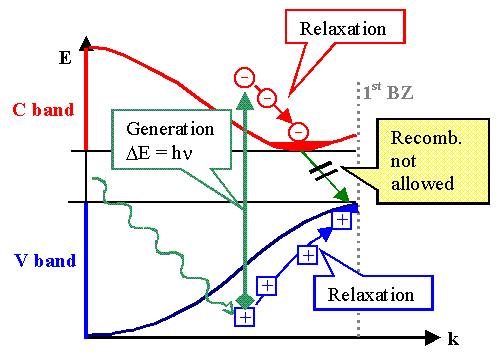Shown is the band diagram for an indirect semiconductor, i.e. the minimum of the conduction band is not directly over the maximum of the valence band.A photon with the energy hn lifts an electron from somewhere in the valence band to a position directly overhead in the conduction band, giving all its energy to the electron. That the new state of the electron lies "directly overhead" is due to the fact that the momentum of the photon is neglibly small.Instant exercise: Show that this is true by estimating the magnitude of the photon's wavevelength, l, for a photon energy of 1 eV. Why is it sufficient to know this value? (Hint: We consider band–band transitions within the first Brillouin zone.)A hole and an electron deep in the valence band or conduction band, respectively, are created which immediately (within picoseconds), by releasing phonons, give up their surplus energy relative to the maximum of the valence band or minimum of the conduction band, respectively, and thus come to an energetic rest at the extrema of the bands.Their wave vectors now are different – direct recombination is not allowed; it would violate Braggs (generalized) law or the conservation of crystal momentum. Recombination needs a third partner (e.g. lattice defects), and life times will be large (typically microseconds if not milliseconds) and depends somehow on the density of suitable third partners.Did you look closely? Yes? Closely enough to notice that this picture contains a very basic mistake (that doesn't influence what has been discussed, however)? Good! If you didn't notice, you may want to activate the link.For direct semiconductors the diagram would be very similar, except that the extrema of the bands are now on top of each other.Recombination now is easily possible, the energy liberated will be in the form of a photon – recombination thus produces light.Life times in the case of direct semiconductors thus tend to be short – typically nanoseconds – and are dominated by the properties of the semiconductor itself.What exactly determines the life time in indirect semiconductors like Si? In our simplified view of "perfect" crystals, would recombination simply be impossible?There are several mechanisms that make recombination possible in real crystals. Generally, a third partner is needed to provide momentum conservation (and is thus also involved in the energy transfer).Usually, this third partner is a defect of some kind. Most notorious are certain atomic defects, often interstitial atoms as, e.g., Fe, Ni, Cu, Au, and many others. But the doping atoms and coarser defects like dislocations and grain boundaries also help recombination along. Last, but also least, it is also possible that phonons get involved.In summary, the life time of indirect semiconductors is dominated by defects, by impurities, by anything that makes the crystal imperfect. It is thus a property that can vary over many orders of magnitude – some examples can be found in the link. In very perfect indirect semiconductors, however, it is a very large time (for electrons), and can easily be found in the millisecond range.

GeneralizationIn an important generalization of what has been said so far, we realize that a minority carrier does not "know" how it was generated. Generation by a thermal energy fluctuation in thermal equilibrium or generation by a photon in non-equilibrium – it's all the same!The minority carrier, once it was generated, will recombine (on average) after the life time t. This is valid for all minority carriers (at least as long as their density is not too far off the the equilibrium value).This implies that the minority carriers will disappear within fractions of a second after they were generated!However, since we have a constant density in thermal equilibrium, we are forced to introduce a generation rate G for minority carriers that is exactly identical to the recombination rate R in equilibrium.In other words, the carrier densities in the valence and conduction bands are not in static, but in dynamic equilibrium (see the thermodynamics script as well).Their (average) density stays constant as long as G = R. Think of your bank account. Its average balance will be constant as long as the withdrawal rate is equal to the deposition rate.From the values of the densities we can not make any statement as to the recombination and generation rate – your bank balance stays constant if you withdraw and deposit 1 \$ a week or 1 million \$!If we know the life time t, however, we can immediately write down the recombination rate:R is the number of recombinations per second (and per cm3), i.e. R = nmin/t with nmin = density (or number; as always we use these quantities synonymously even so this is not strictly correct) of minority carriers.With the relations from above we obtain the following expression:
G  =  R  =  ni2
t · NDop
With NDop = density of the doping atoms.This equation is a good approximation for the temperature range where the doping atoms are all ionized but little band-band transitions take place, i.e. in the temperature range where the semiconductor is usually used. And do not forget: it is only valid in thermodynamic equilibrium.

Diffusion LengthMinority (and majority) carriers are not sitting still in the lattice (they are only "sitting still" in k-space!), but move around with some average velocity.In the free electron gas model this velocity could be directly calculated from the momentum mv =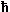·k, so v =k/m.But this is the phase velocity and this relation does not apply to the holes and electrons at the edges of the valence and conduction band, i.e. in the region of the dispersion relation where the differences between the free electron gas model and the real band structure is most pronounced and the group velocity can be very low if not zero.For the purpose of this subchapter let's simply assume that the carriers move randomly through the lattice (being scattered all the time) and that this movement can just as well be described by a diffusion coefficient D as always.We already had a glimpse that there is a connection between the mobility µ and the random movement of the carriers. Here we will take this for granted (we will come back to this issue later) and take note of this simple, but far-reaching relation, called Einstein relation . This relation connects the mobility and the diffusion coefficient D via
D  =  µ · kT
eThe diffusion coefficient D, of course, is the proportionality constant that connects the diffusion current j of particles (not necessarily an electrical current!) to their density gradient Ñr(x,y,z) (with r = density of the particles) via Fick's first law:
j(x,y,z)  =  –  D · Ñr(x,y,z )Bearing this in mind, we now can relate the average distance = (x2 + y2 + z2)1/2 that a particle moved away from its position (0,0,0) at t = 0 after it diffused around for a time t via another Einstein relation by
  =  D 1/2 · t æ è ö ø æ ç è ö ÷ øA minority carrier with a specific life time t thus will be found at an average distance from the point were it was generated given by
  = æ è ö øThis specific distance we call the diffusion length L of the minority carriers.The diffusion length L is just as good a measure of the dynamics of the carrier system as the life time t ; we can always switch from one to the other via the Einstein relations.Long life times correspond to long diffusion length. In todays Si, diffusion lengths of mm can be achieved – a tremendous distance in the world of electrons and holes.

 Real SemiconductorsSo far we have explicitly or implicitly only considered "perfect" semiconductors – i.e. semiconductors without any unintentional lattice defects. But now it is easy to consider real semiconductors. Real semiconductors contain unintentional lattice defects and these defects can have two major detrimental effects if they introduce energy levels in the band gap.They may influence or even dominate the carrier density, i.e. they act as unwanted dopants. In many cases of semiconducting materials without any technical applications so far, the carrier density is pretty much determined by defects and is practically unalterable (it usually also comes in only one kind of conductivity type).They influence or dominate the minority carrier life time. This may be a major problem, e.g. for solar cells where large life times are wanted in rather imperfect materials.In addition, they will also influence the mobility (usually decreasing it).Technically, there are several options for dealing with real semiconductors.Make the material very perfect. This is the recipe for Si microelectronics and most of the III-V compound devices.Live with the defects and render them impotent as far as possible, e.g. by hydrogen passivation.Find semiconductors where the defect levels are not in the band gap (after passivation). An example may be CuInSe2, which is usable for solar cells despite lots of defects, and GaN, which is the standard material for blue light-emitting diodes.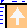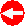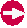© H. Föll (Semiconductors - Script)